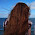## Group Anagrams

Given an array of strings, group anagrams together.

For example, given: ["eat", "tea", "tan", "ate", "nat", "bat"]
Return:

[
["ate", "eat","tea"],
["nat","tan"],
["bat"]
]

Note: All inputs will be in lower-case.

## Analysis:

In the problem of anagram, hash map is quite often used to efficiently get us to the correct answer.
For this specific quesion, the key is how we set the key to the map so that for each str in the array, the key can be efficiently computed.

One slower version is to use the sorted string as the key, and for each string  a sort operation is required to compute the key. The complexity is O(n * m log m) where n is the number of strings, and m is the length of each string.

However, another way of compute the key is more efficient, we could speed up the complexity to O(n * m).

Thinking about the prime numbers, for each char in the string, we can compute the multiplication for one prime number corresponding to the char. In such way, only anagram strings become the same number.  For the prime numbers, we can just precompute since there are only 26 prime numbers we need.

### Slow Code (C++):

class Solution {
public:
vector<vector<string>> groupAnagrams(vector<string>& strs) {
vector<vector<string>> res;
map<string, vector<string> > mp;
for (int i=0;i<strs.size();++i){
string key = strs[i];
sort(key.begin(), key.end());
if (mp.find(key) == mp.end()){
mp[key] = vector<string>(1,strs[i]);
}else{
mp[key].push_back(strs[i]);
}
}
for (auto it = mp.begin(); it !=mp.end(); it++){
res.push_back(it->second);
}
return res;
}
};

## Code (C++):

class Solution {
public:
vector<vector<string>> groupAnagrams(vector<string>& strs) {
vector<vector<string>> res;
int primes[]={2, 3, 5, 7, 11, 13, 17, 19, 23, 29, 31, 37, 41, 43, 47, 53, 59, 61, 67, 71, 73, 79, 83, 89, 97, 101};
map<int, int> mp;
int key;
int count = 0;
for (int i=0; i<strs.size();++i){
key = 1;
for (int j=0; j < strs[i].size();++j){
key *= primes[strs[i][j]-'a'];
}
if (mp.find(key) == mp.end()){
mp[key] = count++;
res.push_back(vector<string>(1, strs[i]));
}else{
res[mp[key]].push_back(strs[i]);
}
}
return res;
}
};

## Code(Python):

class Solution(object):
def groupAnagrams(self, strs):
"""
:type strs: List[str]
:rtype: List[List[str]]
"""
primes = [2, 3, 5, 7, 11, 13, 17, 19, 23, 29, 31, 37, 41, 43, 47, 53, 59, 61, 67, 71, 73, 79, 83, 89, 97, 101]
mp = {}
res = []
count = 0
for str in strs:
key = 1
for ch in str:
key *= primes[ord(ch) - ord('a')]
if mp.get(key) is None:
mp[key] = count
res.append([str])
count += 1
else:
res[mp[key]].append(str)
return res

1.Hello There,

I learnt so much in such little time about leetcode Question: Group Anagrams. Even a toddler could become smart reading of your amazing articles.

I just wanted to know How can we use WebSQL ?

I look forward to see your next updates.

Kind Regards,
Preethi

2.Greetings Mate,

Brilliant article, glad I slogged through the #topic it seems that a whole lot of the details really come back to from my past project.

why is saying c programming is a based on java?

Very useful article, if I run into challenges along the way, I will share them here.